##### Pre-Calculus All-in-One For Dummies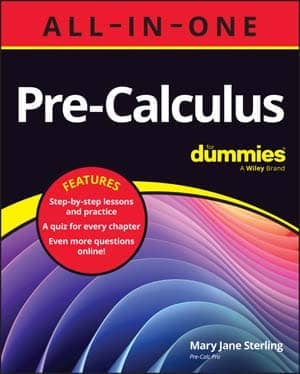Logarithmic equations take different forms. As a result, before solving equations that contain logs, you need to be familiar with the following four types of log equations:

• Type 1. In this type, the variable you need to solve for is inside the log, with one log on one side of the equation and a constant on the other. Turn the variable inside the log into an exponential equation (which is all about the base, of course). For example, to solve log3 x = –4, change it to the exponential equation 3–4 = x, or 1/81 = x.

• Type 2. Sometimes the variable you need to solve for is the base. If the base is what you’re looking for, you still change the equation to an exponential equation. If logx 16 = 2, for instance, change it to x2 = 16, in which case x equals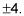Keep in mind that because logs don’t have negative bases, you throw the negative one out the window and say x = 4 only.

• Type 3. In this type of log equation, the variable you need to solve for is inside the log, but the equation has more than one log and a constant. You can solve equations with more than one log. To solve log2(x – 1) + log2 3 = 5, for instance, first combine the two logs that are adding into one log by using the product rule: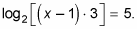Turn this equation into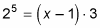to solve it. The solution is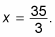• Type 4. What if the variable you need to solve for is inside the log, and all the terms in the equation involve logs? If all the terms in a problem are logs, they have to have the same base in order for you to solve the equation. You can combine all the logs so that you have one log on the left and one log on the right, and then you can drop the log from both sides. For example, to solve log3(x – 1) – log3(x + 4) = log3 5, first apply the quotient rule to get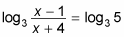You can drop the log base 3 from both sides to get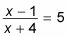which you can solve easily by using algebra techniques. When solved, you get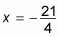Keep in mind that the number inside a log can never be negative. Plugging this answer back into part of the original equation gives you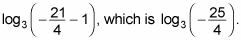You don’t even have to look at the rest of the equation. The solution to this equation, therefore, is actually the empty set: no solution.

Always plug your answer to a logarithm equation back into the equation to make sure you get a positive number inside the log (not 0 or a negative number).